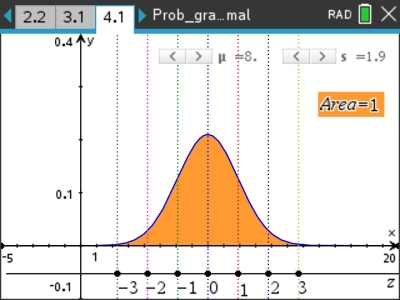# Activities

•Aust Senior

1 Hours

• ##### Device
•TI-Nspire™ CX CAS

5.2

## Graphs of Continuous Distributions#### Activity Overview

This activity focuses on graphs of probability density functions for continuous random variables, including the normal and standard normal distributions.

#### Objectives

The activity helps highlight the difference between discrete and continuous probability distributions, in part by taking a calculus approach to various calculations.

#### Vocabulary

• Mean
• Variance / Standard Deviation
• Integral Calculus